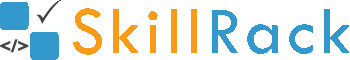Sum of Digits in a Number2 months ago

The program must accept an integer N as the input. The program must print the sum of all the digits in N as the output.

Boundary Condition(s):
1 <= N <= 10^8

Input Format:
The first line contains N.

Output Format:
The first line contains the sum of all the digits in N.

Example Input/Output 1:
Input:
12345

Output:
15

Explanation:
The sum of all the digits in 12345 is 15.
Hence the output is 15

Example Input/Output 2:
Input:
5000

Output:
5

The below video explains the logic and the implementation in C programming language to find the sum of digits in a number.

#include <stdio.h>
#include <stdlib.h>

int main()
{
int N;
scanf("%d", &N);
int sum = 0;
while(N != 0)
{
sum = sum + N%10;
N = N/10;
}
printf("%d", sum);
return 0;
}

#include <iostream>

using namespace std;

int main()
{
int N;
cin >> N;
int sum = 0;
while(N != 0)
{
sum = sum + N%10;
N = N/10;
}
cout << sum;
}

import java.util.Scanner;

public class Hello {

public static void main(String[] args) {
Scanner sc = new Scanner(System.in);
int N = sc.nextInt();
int sum = 0;
while (N != 0) {
sum = sum + N % 10;
N = N / 10;
}
System.out.print(sum);
}

}

N = int(input())
sumOfDigits = 0
while N != 0:
sumOfDigits += N%10
N //= 10
print(sumOfDigits)